# Energy Potential Kinetic Energy Forms Energy Energy is

• Slides: 27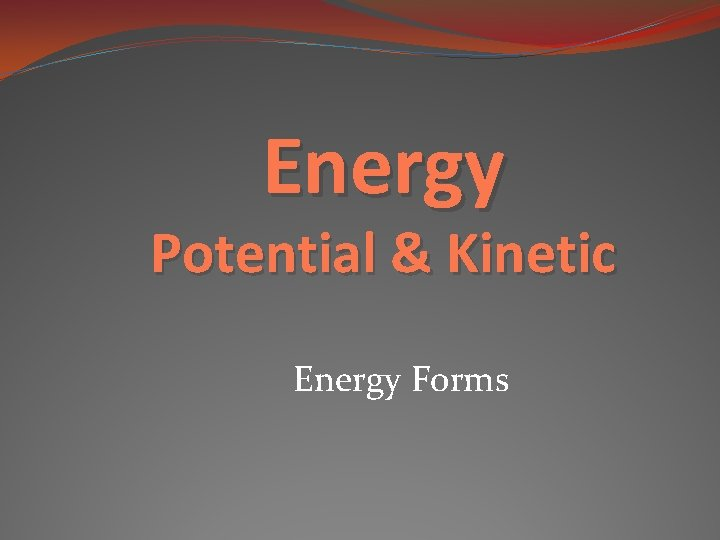Energy Potential & Kinetic Energy Forms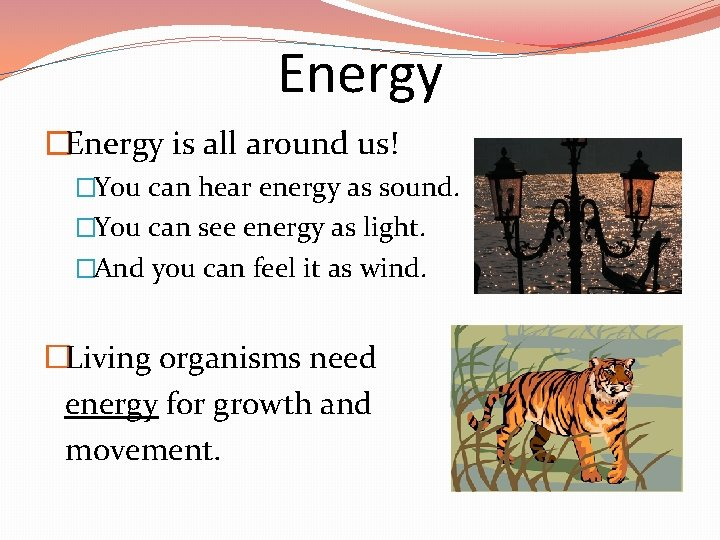Energy �Energy is all around us! �You can hear energy as sound. �You can see energy as light. �And you can feel it as wind. �Living organisms need energy for growth and movement.What is Energy? �Energy- means the ability to do work. �The SI unit for energy is Joules ( J ); same as work. �Remember that work is exerting a force that causes an object to move.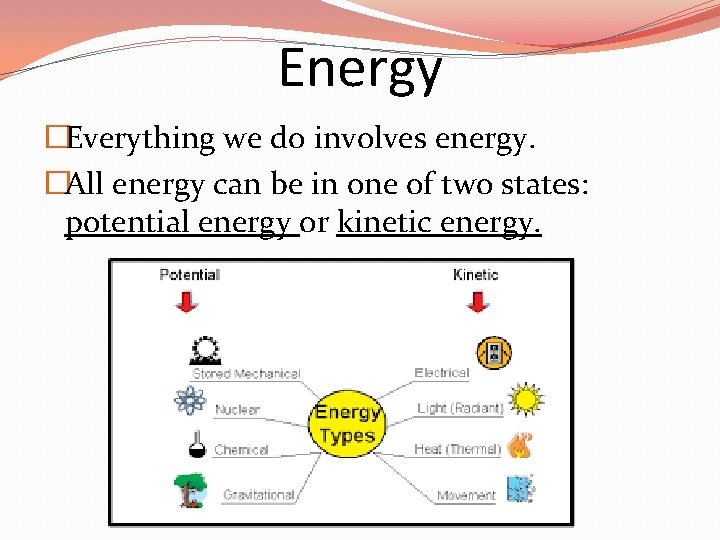Energy �Everything we do involves energy. �All energy can be in one of two states: potential energy or kinetic energy.Potential Energy �Potential energy is stored energy due to an object’s position--energy ready to go. �Ex: a drawn bow �Ex: a car on top of a hill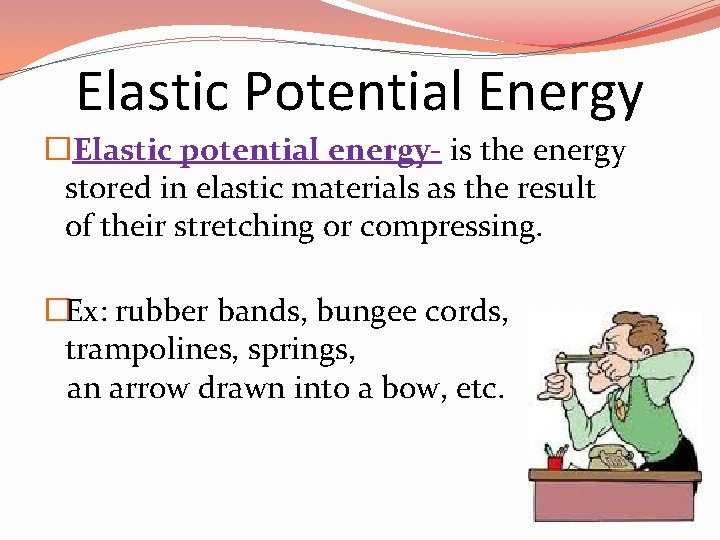Elastic Potential Energy � Elastic potential energy- is the energy stored in elastic materials as the result of their stretching or compressing. �Ex: rubber bands, bungee cords, trampolines, springs, an arrow drawn into a bow, etc.Gravitational Potential Energy �Potential energy that is related to an object's height above the ground is known as gravitational potential energy. �Ex: A waterfall, a suspension bridge, and a falling snowflake.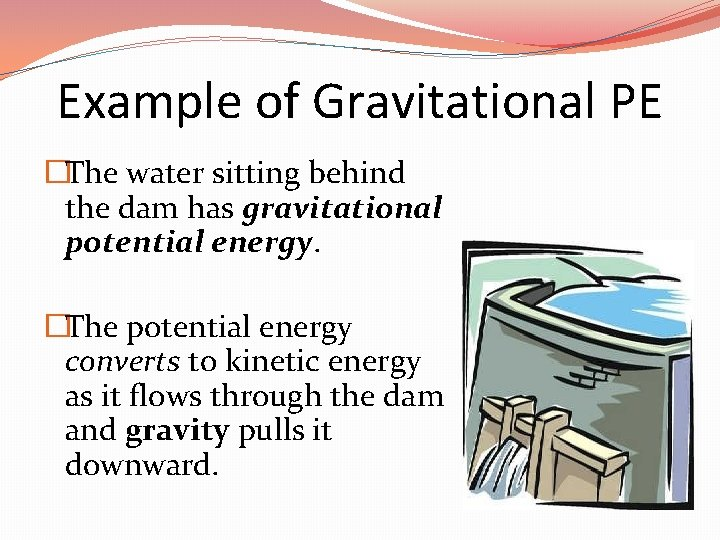Example of Gravitational PE �The water sitting behind the dam has gravitational potential energy. �The potential energy converts to kinetic energy as it flows through the dam and gravity pulls it downward.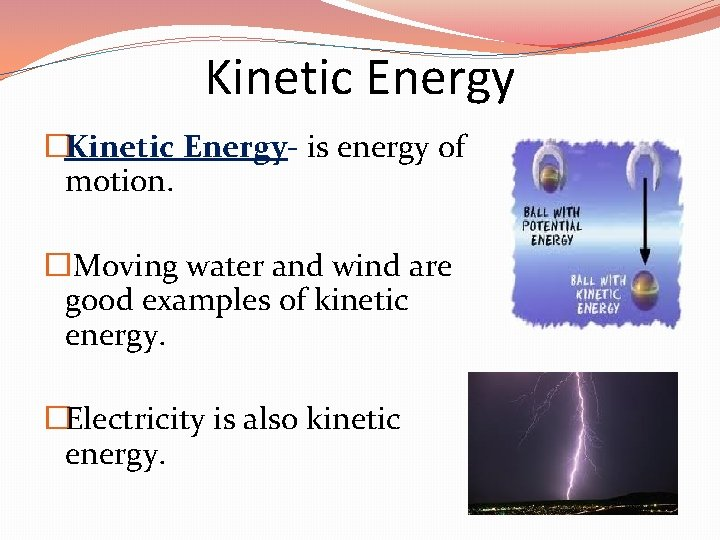Kinetic Energy �Kinetic Energy- is energy of motion. � Moving water and wind are good examples of kinetic energy. �Electricity is also kinetic energy.Kinetic Energy �The faster an object moves, the more kinetic energy it has. �The greater the mass of a moving object, the more kinetic energy it has.Kinetic energy slows as Potential energy gains Kinetic energy Maximum as it leaves hand. Potential energy decreases as Kinetic energy increases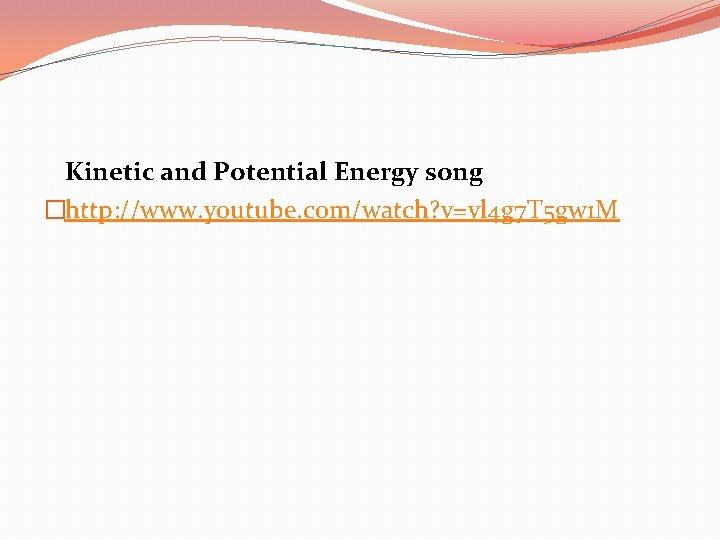Kinetic and Potential Energy song �http: //www. youtube. com/watch? v=vl 4 g 7 T 5 gw 1 M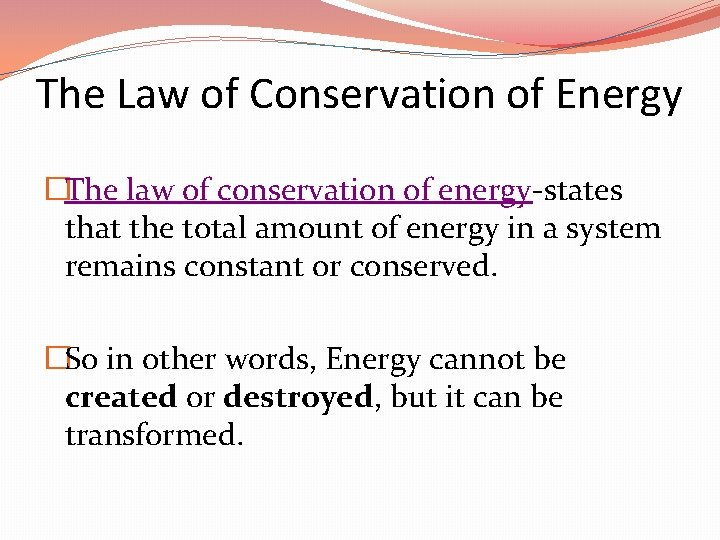The Law of Conservation of Energy �The law of conservation of energy-states that the total amount of energy in a system remains constant or conserved. �So in other words, Energy cannot be created or destroyed, but it can be transformed.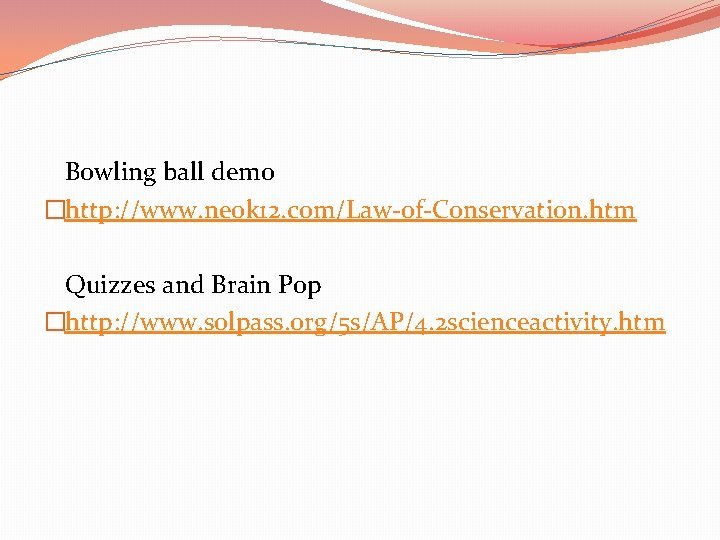Bowling ball demo �http: //www. neok 12. com/Law-of-Conservation. htm Quizzes and Brain Pop �http: //www. solpass. org/5 s/AP/4. 2 scienceactivity. htmCalculating Kinetic Energy �Formula: KE= ½ mv² (1/2 x mass x velocity²) �Ex. 1: Determine the kinetic energy of a 625 kg roller coaster car that is moving with a velocity of 18. 3 m/s. �Ex. 2: What is the KE of a soccer ball which has a mass of 0. 8 kg and is kicked at a velocity of 10 m/s?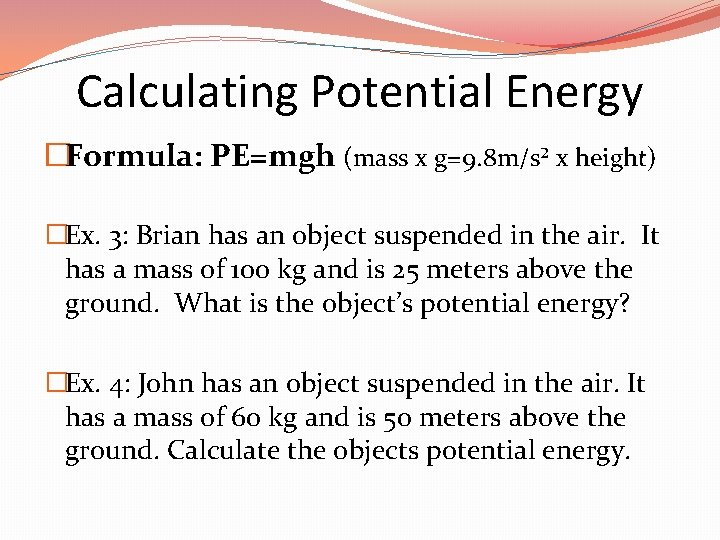Calculating Potential Energy �Formula: PE=mgh (mass x g=9. 8 m/s² x height) �Ex. 3: Brian has an object suspended in the air. It has a mass of 100 kg and is 25 meters above the ground. What is the object’s potential energy? �Ex. 4: John has an object suspended in the air. It has a mass of 60 kg and is 50 meters above the ground. Calculate the objects potential energy.Energy Forms Energy Transformations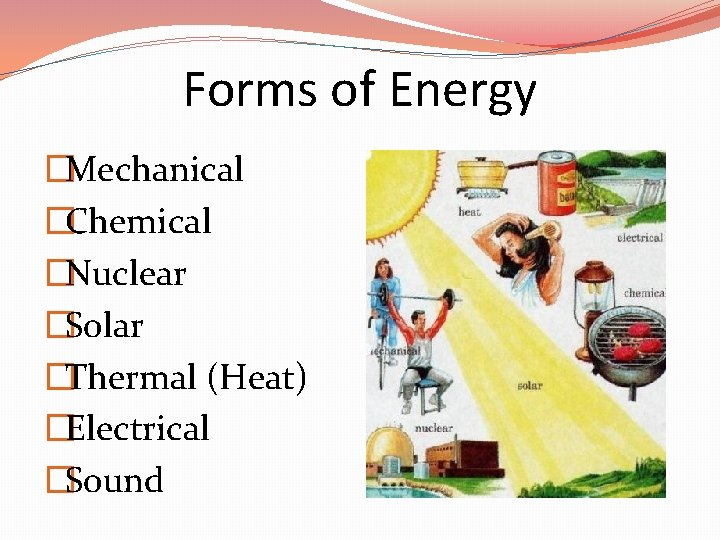Forms of Energy �Mechanical �Chemical �Nuclear �Solar �Thermal (Heat) �Electrical �Sound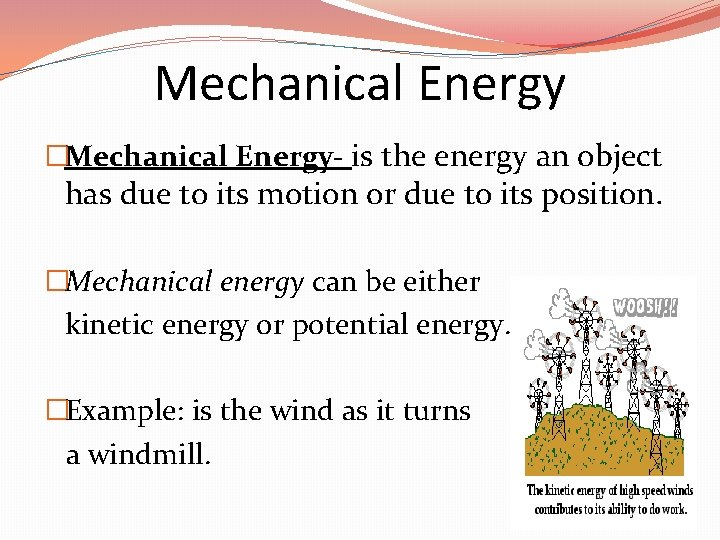Mechanical Energy �Mechanical Energy- is the energy an object has due to its motion or due to its position. �Mechanical energy can be either kinetic energy or potential energy. �Example: is the wind as it turns a windmill.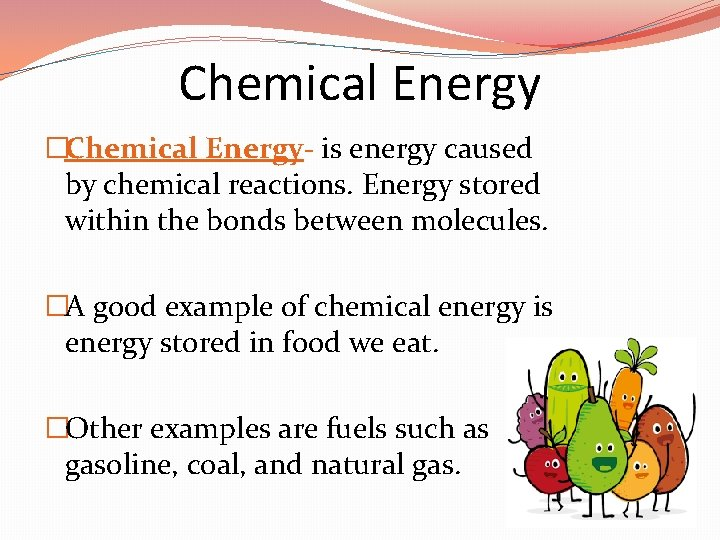Chemical Energy �Chemical Energy- is energy caused by chemical reactions. Energy stored within the bonds between molecules. �A good example of chemical energy is energy stored in food we eat. �Other examples are fuels such as gasoline, coal, and natural gas.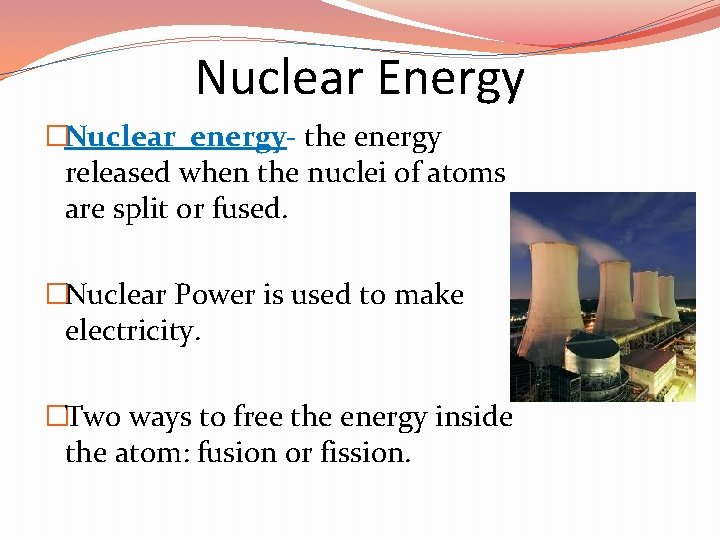Nuclear Energy �Nuclear energy- the energy released when the nuclei of atoms are split or fused. �Nuclear Power is used to make electricity. �Two ways to free the energy inside the atom: fusion or fission.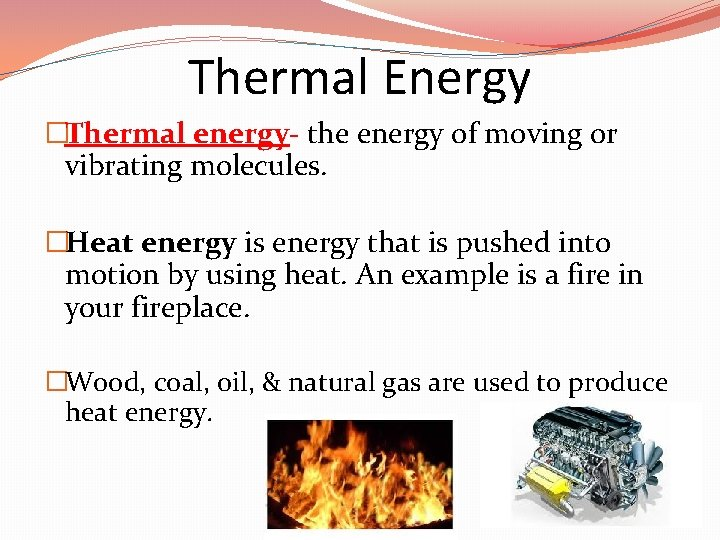Thermal Energy �Thermal energy- the energy of moving or vibrating molecules. �Heat energy is energy that is pushed into motion by using heat. An example is a fire in your fireplace. �Wood, coal, oil, & natural gas are used to produce heat energy.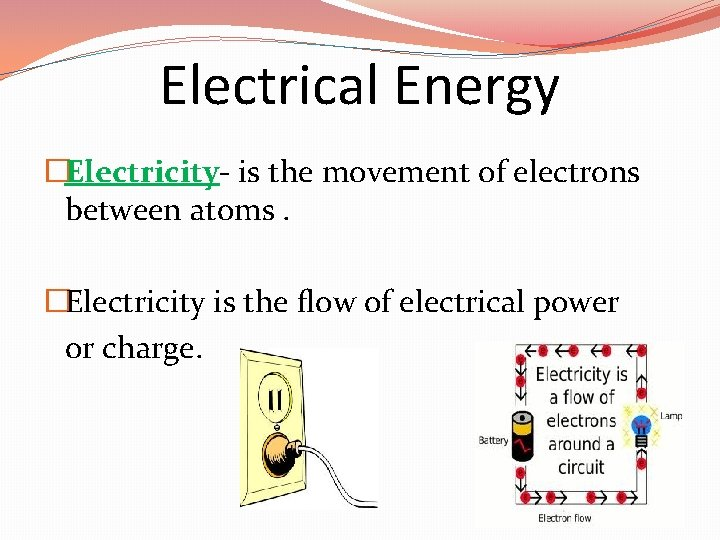Electrical Energy �Electricity- is the movement of electrons between atoms. �Electricity is the flow of electrical power or charge.Electrical Energy Examples of electrical energy: �electric coils on your stove � light bulbs � batteries � lightning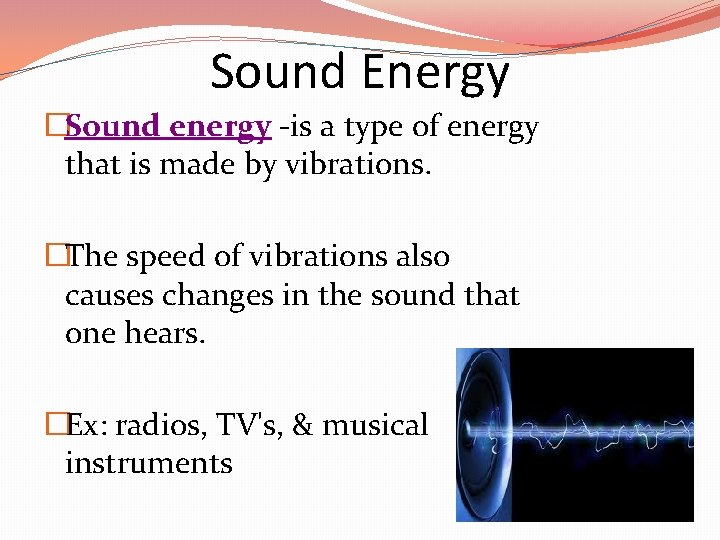Sound Energy �Sound energy -is a type of energy that is made by vibrations. �The speed of vibrations also causes changes in the sound that one hears. �Ex: radios, TV's, & musical instruments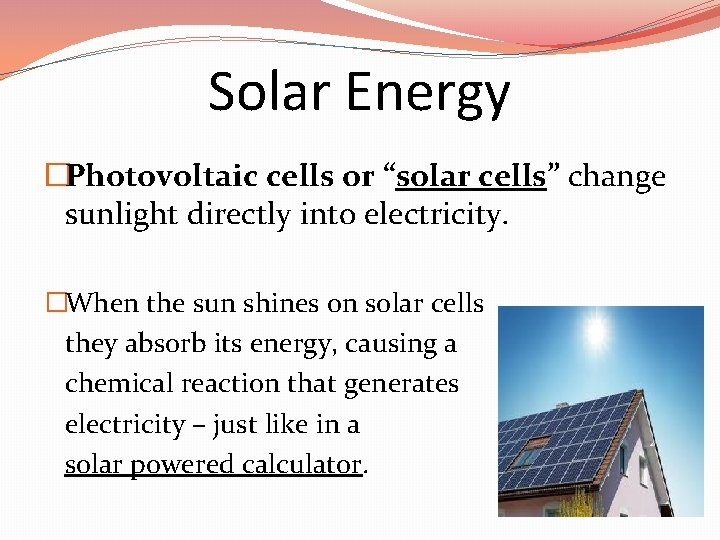Solar Energy �Photovoltaic cells or “solar cells” change sunlight directly into electricity. �When the sun shines on solar cells they absorb its energy, causing a chemical reaction that generates electricity – just like in a solar powered calculator.Solar Energy �Solar energy -is the sun’s rays (solar radiation) that reach the Earth. �Solar energy can be converted into other forms of energy, such as heat and electricity.